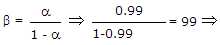# Electronics and Communication Engineering - Electronic Devices and Circuits - Discussion

5.

A transistor has a current gain of 0.99 in the CB mode. Its current gain in the CC mode is

 [A]. 100 [B]. 99 [C]. 1.01 [D]. 0.99

Explanation:Current gain = 1 + β = 100.

 Rakesh said: (Feb 1, 2015) Sir I don't know about CB and CC please tell me briefly?

 Kamran Saifi said: (Feb 3, 2015) CB is Common Base & CC is Common Collector mode. The current gain in CB mode is Ic/Ie & for CC mode it is Ic/Ib.

 Nandan Kumar said: (Feb 17, 2015) Please tell me about CB mode is Ic/Ie & CC mode is Ic/Ib.

 R.Gayathri said: (Jul 14, 2015) It was good give brief explanation.

 Dilip said: (Sep 7, 2015) CB = ic/ie. CC = ic/ib.

 Hussain said: (Nov 10, 2015) Ic/ie = Alpha. Ic/ib = beta, b = a/(1-a). A given = 0.99. So b = 99 = 1+99 = 100.

 Billz said: (Dec 26, 2015) Why are you adding 1 to 99?

 Ajay P Roy said: (May 31, 2016) In CB config: CURRENT GAIN (ALPHA, a) : Ic/Ie=.99 (given). In CE config: CURRENT GAIN (BETA, b) : Ic/Ib. In CC config: CURRENT GAIN (gamma, g) : Ie/Ib. And b = a/(1 - a). Then, g = 1 + b. Therefore: g = 1 + (a/(1 - a)). g =1/(1 - a) = 1/(1 - .99) = 1/.01 = 100.

 Gouarv said: (Jan 17, 2017) Very helpful, Thanks @Ajay P Roy.

 Sai Kumar Kunchala said: (Apr 27, 2017) Excellent explanation Thanks, @Ajay.

 Pallavi Roy said: (Jun 7, 2017) Clearly explained, Thanks @Ajay.

 Ankesh said: (Jul 22, 2017) Thanks @Ajay!

 K V Satyanarayana said: (Aug 30, 2017) Thanks @Ajay.

 Chandra said: (Sep 12, 2017) Thanks @Ajay.

 Venky said: (Oct 28, 2017) Thanks @Ajay.

 Gurunadham said: (Nov 27, 2017) γ=1+β=α/(1-α).

 Chaitanya said: (Dec 11, 2017) Thanks @Ajay.

 Faizi said: (Feb 18, 2018) Thanks @Ajay.

 Monika said: (Feb 22, 2018) Thanks a lot @Ajay.

 Ashvani Jha said: (Feb 26, 2018) Please explain about CB and CC.

 Pawan said: (Apr 11, 2018) Thanks @Ajay.

 Saravana said: (Apr 23, 2018) The explanation is super, Thanks @Ajay.

 Sreeju said: (Jul 19, 2018) Γ=1÷(1-α).

 Nimrod said: (Aug 5, 2018) Why do we change modes from CC to CB or vice versa? What is the practical application of changing transistor modes?

 Nallendran said: (Feb 24, 2019) Thanks for the answer @Ajay.

 Jyoti Prasad said: (Nov 12, 2019) Y-> gama B-> beta < ---> alpha Y = B+1 = 1/1- < = 1/(1-0.99) = 100.

 Satyam said: (Dec 9, 2019) Very clearly explained thanks @Ajay.